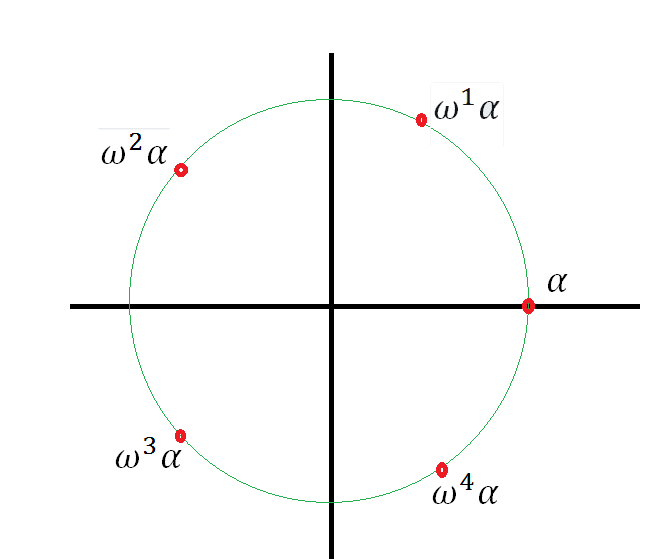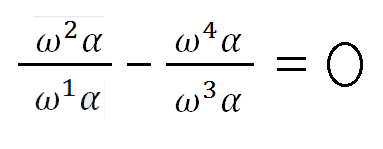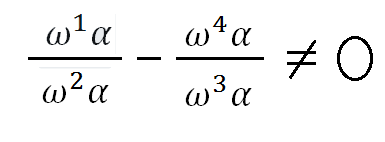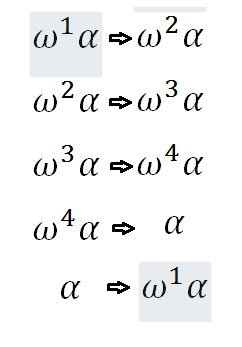## Tuesday, September 11, 2012

### How can you shuffle the fifth roots of two?

In my last post, I talked about how you can generate new types of numbers by taking square roots (or higher roots) of numbers you already have. You can try and think of more intricate things you might do, like complicated expressions involving sums and quotients, but it turns out that it is sufficient to worry only about expressions where you take roots of things you already have.

On the other hand, the universe of algebraic numbers which actually exist is made up of those numbers which are the solution of algebraic equations. The question becomes: can the members of this set be generated through our constructive mechanism of adjoining roots to fields which we have already created by adjoining roots to smaller fields? We will try to answer this question by looking at the permutation properties of algebraic numbers.

We can show that in the most general case, the roots of a fifth degree equation are indistinguishable from one another: you can swap one for another any which way, and any statement which started out being true will still be true after an arbitrary reshuffling. Of course, we can also find particular fifth-degree equations for which this fails to hold true. But if we are to hope to solve a general fifth-degree equation, then we must expect that we will be able to write an expression which takes on five different values; and that we are furthermore free to shuffle those values about with impunity, always yielding consistently true or false results when resolved to a rational expression.

We remarked last time that for the case of the third degree equation, the three cube roots of two met this requirements. The truth or falsehood of an algebraic expression would not be altered by any arbitrary re-shuffling of the cube roots of two. We have to ask: do we get a similar situation with the fifth roots of two?

We do not. Here is a map showing the location of the fifth roots of two in the complex plane:You can see that I have taken alpha to be the real fifth root of two, and omega to be a fifth root of unity. You remember when we talked about the cube roots of two, I said we could swap them about any which way and not encounter any contradictions. Well, it doesn't quite work that way with the fifth roots. Suppose we swap, for example, the the two "positive" complex roots, which I'll abbreviate in text as w1 and w2. Now, before swapping them, I could have written:It's true because the quotient of any two consecutive roots is just omega. But it's no longer true if I swap w1 and w2:So whatever may be the permutabilities of the fifth roots of two, they are not without some restriction. In fact, from the present example, we can see quite clearly that if we change w1 to w2, the following changes must all follow in due course in order that the quotients maintain their equality:If you apply this permutation five times in a row, you get back to the original arrangement. By the way, it's important to point out that I haven't shown that this is an allowed permutation of the roots. It's possible that the permutation shown here leads to inconsistent results when you plug the altered roots into some particular equation. All I've shown is that this permutation is not specifically ruled out by the taking of quotients.

Although I'm not going to prove it today, it will turn out that this cycle of permutations is indeed valid for the fifth roots of two. But these are not the only permissible re-shufflings. It goes without saying that complex conjugates can always be swapped with each other. So we can flip the whole circle over the horizontal axis. When we compound this with the rotations, that gives us a total of ten reshufflings. I believe that this is called the "dihedral group on five elements".

Does that complete our description of the Galois group of this particular algebraic field? Not quite. There are other allowed permutations, and they are inherited from the permutation properties of the fifth roots of unity. But we'll take that up another day.

#### 1 comment:Anonymous said...

http://m.wolframalpha.com/input/?i=x%5E6-2%3D0&x=0&y=0

x^n-2=0

plug in x^n=x^n * e^(i 2 pi m) where m are integers n times arround the circle .

x=2^(1/n)*e^(- i 2 pi m/n)

Is the solution.